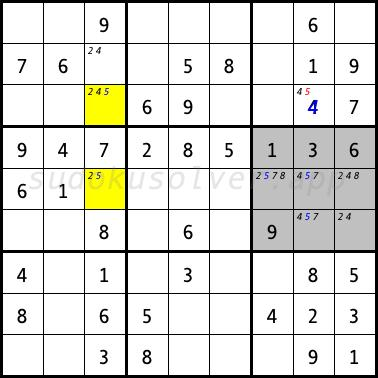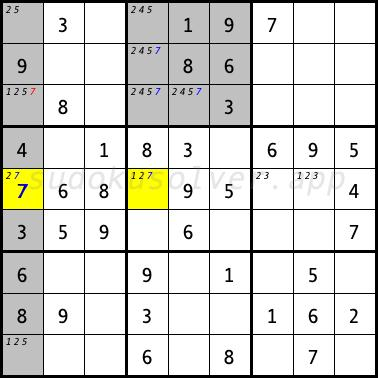# Empty Rectangle

An Empty Rectangle occurs when 4 cells in a box form a rectangle and do not contain a given digit. Thus, the remaining cells of this box, which may contain the given numberr, lie in one column and in a row. In the following sudoku diagram for the digit 5, an empty rectangle appears in the right middle box in the cells R4C7, R4C9, R6C7 and R6C9. The cells that can have the digit 5 are only in the fifth row and the eighth column. An empty rectangle alone is not enough to carry out the elimination. In the third column, only two cells R3C3 and R5C3 might have the digit 5. If the cell R5C3 has the digit 5, then in the right middle box, the cells that may get this digit are limited to the eighth column. Thus, the R3C8 cell will not have the digit 5. If the R3C3 cell has the digit 5, the R3C8 cell will not get this digit again. Thus, the candidate 5 can be eliminated from the R3C8 celll. After applying this elimination, this cell can only have the digit 4.In the following sudoku diagram for digit 7, an empty rectangle appears in the top middle box. It is formed by the R1C5, R1C6, R2C5 and R2C6 cells. Cells that can have the digit 7 lie only in the third row and fourth column. In the fifth row, only two cells R5C1 and R5C4 can have the digit 7. If the cell R5C4 has the digit 7, then in the middle top box, the cells that can have this digit are limited to the third row. Thus, the R3C1 cell will not have the digit 7. If the R5C1 cell has the digit 7, the R3C1 cell will not get the value again. Thus, the digit 7 can be eliminated from the R3C1 cell. After this elimination, the digit 7 can only be place in the R5C1 field in the first column.